# Monthly Archives: October 2013

In the previous blog, I generated a graph to show that a constant voltage active load driven by a high impedance source (in this case 240 volts RMS 50 Hz supply in series with a 3 Henry inductor) could establish one of two voltages to draw a particular power (in this case 18 watts). Here is the graph.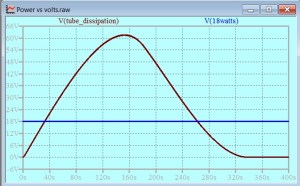See the previous post for details. I really wanted to show this on a graph with current vs. Voltage axes, where the constant power line would be an hyperbola and it would cross the load line in two places. Here I set out to do this.

Readers of this blog are likely to be familiar with the notion that the vector voltage across a resistor, and the vector voltage across a reactance in series, provide us with the wherewithal to construct a circular locus. In reflecting on this task, I have realized that in fact there are two different circles, and that we choose the one we want according to context. In small signal circuits, the reactance is most often a capacitor, but an inductive reactance shares the same critical property: the vector voltage is at right angles with the vector voltage across a resistor in series. Consider this circuit: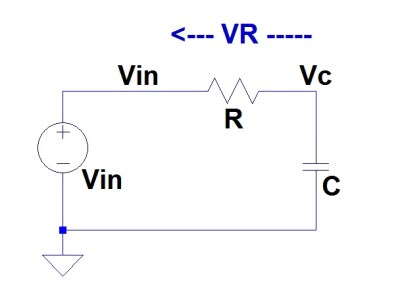The voltage across the resistor, VR and the voltage across the capacitor VC add to give the input voltage Vin. (Kirchhoff, or something, isn’t it?) Vectorially, the addition of voltages can be represented thus: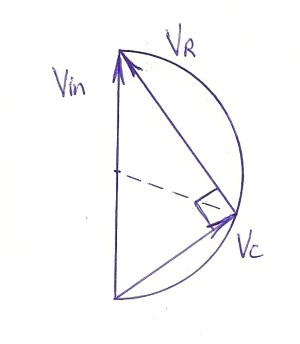As we learned in high school maths, “The angle in a semicircle is a right angle”. This vector (phasor) diagram is lovely to reflect on. It does not matter what the relative proportions of VR and VC are, the radius (shown dashed) of the circle to the point of connection between the vectors is constant. The relationship between the proportions of the vectors might vary with frequency as the reactance varies with frequency: here we have the genisis of an all-pass filter. On the other hand, if the radius is held fixed and at right angles to the input voltage by feedback, we have half a bridge that might form the basis of an audio oscillator.

I realized that if the supply was a constant AC voltage with an inductor in series, then a circular or elliptical load line would arise for a resistive load.Such a circle, (or an ellipse, which is just a circle with different scales in the orthogonal directions) does turn up on a load line for a source that has a reactive load. Here is a diagram from the Radiotron Designer’s Handbook – this is a loadline for instantaneous values: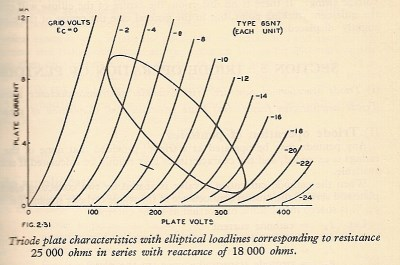This elliptical load line is for a resistance and a reactance in series. Exactly the same ellipse would be traversed by a capacitative reactance and an inductive reactance, but the instantaneous voltage on the anode of that triode would follow the ellipse in different directions in the two cases (anti-clockwise for a capacitor, and clockwise for an inductor).

The semicircle shown in the first diagram might have seemed to be defined (in part) by the relative angles of the vectors. However the same triangle could have been constructed with lines representing the magnitudes of scalars, such as the RMS values of voltages. Here is where we discover that there are two different circles generated by the very same vectors.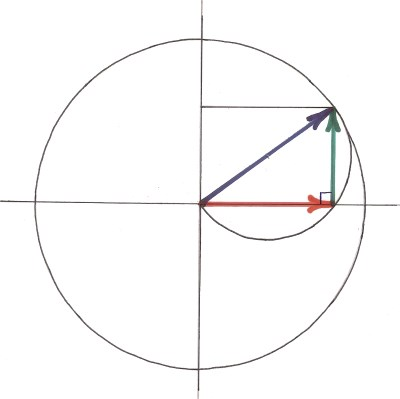Here, the supply voltage (blue vector) is shown as the sum of the resistor volt drop (red) and the inductor volt drop (green). The semicircle that we considered above is shown with (as above) the supply voltage being a diameter. If we show the vectors on Cartesian coordinates where the resistive drop and the inductive drop are shown on some axes, rather than just in free space and not relating to anything but each other, we see that the sum of them falls on a circle centered at the origin, and that the sum (the input voltage) is a radius of this circle.

This representation can be applied to RMS values, even though they are scalars. We can form the triangle with vectors with prior knowledge that the inductor volt drop and the resistor volt drop can be represented as vectors at right angles. Once we have the magnitudes of Input Volts, Inductor Volts, and Resistor volts, we can place lines representing them on “Inductor Volts vs Resistor Volts” axes, and produce a triangle that is similar (in the formal sense) to the vector one. If we normalize the inductive reactance to the value 1 then the inductor will pass one amp for every volt across it. The above circle diagram can then represent the inductor current (which is the same as the resistor current for a series circuit, or just “The current”) on the vertical axis, and the resistor volts on the horizontal axis. The “Load Line” for the high impedance supply is then a quarter of the big circle. We can do this without normalizing the inductive reactance, but the curve will then be an ellipse.

In the last post,(q.v.)  I drew the diagram Tube dissipation vs Volts – (reporoduced at the top of this post). The issue at hand was how the dissipation of a load would vary with the voltage across that load when the supply was a 240 volt RMS voltage provided via a 3H inductor. There were other complications: you would have to read that post. I wanted to see this picture on Current vs. Voltage axes, but the complications made this tricky. Here is a simplified case. the load is resistive. It is connected in series with a 3H inductor to a 240 v RMS supply. This is NOT a loadline for instantaneous values. Each point on these axes represents averaged (RMS is the average chosen) values for the whole cycle of the alternating waveform. Whereas in the instantaneous values loadline (above) the independent variable is the grid voltage, in the graph below, each point on the blue line represents a different value for the resistance.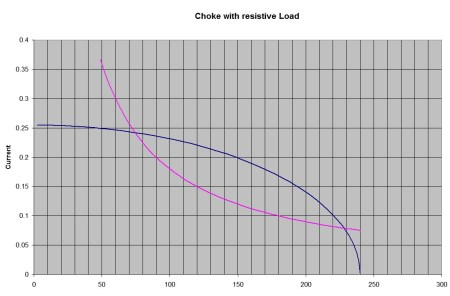The violet line represents a constant power of 18 watts. This is, of course an hyperbola on these axes. The blue line represents the power available to the resistor as the resistance is varied. the left-most intersection of these lines is the point for a low voltage electronic circuit. At about 75 volts in this case.

# Is a skim across the top worse than no analysis at all?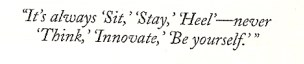Following on from the last post, I wanted to start with a picture again. Who said it was a dog’s life being an engineer?

A young mate of mine, after browsing this blog, suggested that I should make it a movie blog like that of Dave Jones at http://www.eevblog.com/
To be honest, I am not sure how I was supposed to take this. The subject of Dave Jones is too big to deal with in just one post, so I will just report on my latest squint at eevblog here.

The latest posting on eevblog at the time of writing is EEVblog #533 – LED Fluoro Tube Teardown He might have moved on by the time you read this, but you can work your way back through his postings to see this one. When I visited the site, it was sporting an advertisement. I suppose that is how Dave makes a quid out of his eevblog site. (He says it is his full time job.) This particular ad had a picture of a woman with a look on her face like a rabbit that is frozen in the spot light whist the hunter cocks (bad choice of word, perhaps) his gun. Underneath it says in big bold lettering with a blue background “Date Mature Women!” I don’t think that the woman in the picture could be very mature, or she would not have posed for that photo. I think that in this instance, the word “mature” is a code word for the 20 year old reader, and it means “older than you are”.

In the posting, Dave pulls apart a device that incorporates some electronics and a row of LEDs. The whole thing has the physical form of a fluorescent tube, and is designed to plug into a fluorescent tube fitting.

Dave determines that the electronics consists of a bridge rectifier and res caps up front – a cheap ac to dc conversion which usually comes at the price of low power factor. It seems that we have to be assuming that this device will fit in a light fitting that is equipped with a traditional ballast inductor. I suspect that it would not work in an “electronic ballast” fitting. The power factor would probably not be as bad as it looks on first blush, because the ballast makes this a choke input filter, and together with the power factor correction capacitor, it might come out quite well. This is an interesting situation! It is part of Dave’s style that he skates right past interesting points such as this.

What is the inductance of a fluoro tube ballast? Dave doesn’t say, and I could not find the answer anywhere. If you know where this information is to be found, please let me know. I somehow had the idea that the “on” voltage of a tube is about 70 volts. If this is the case then a 37 watt tube might be modelled like this: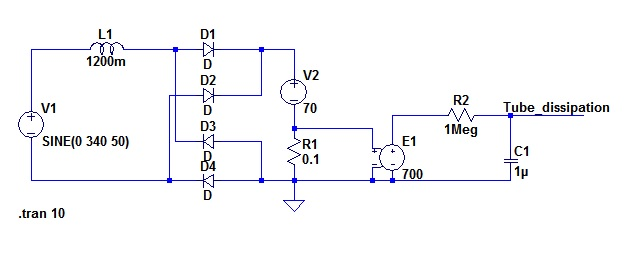240 volt RMS mains is modelled by a sine generator with an amplitude of 340 volts (root 2 times 240)

Of course the real tube will conduct in both directions, but I have modelled it as a bridge rectifier and then a constant voltage source. As the voltage is constant, the power dissipated in the tube is 70 times the current, or 700 times the volt drop in a 0.1 ohm resistor. R2 and C1 give me an average power signal. I have just varied the value of the inductor until I get 37 watts dissipation in the tube. This is a crude model, but it gives me a figure for the inductance to work with – 1200 mH.

The LED light doover that Dave is playing with is advertised as having 18 watts dissipation. There will be two ways of drawing less power from that inductor. One will be with a lower impedance circuit, with a lower voltage and hence less power, and the other with a higher impedance circuit with a lower current and less power. This is the same as saying that the low frequency volts vs. current characteristic of the inductive source will cross the 18 watts hyperbola in two places. Here is a plot of output power vs the voltage at which the energy is taken from the rectifier.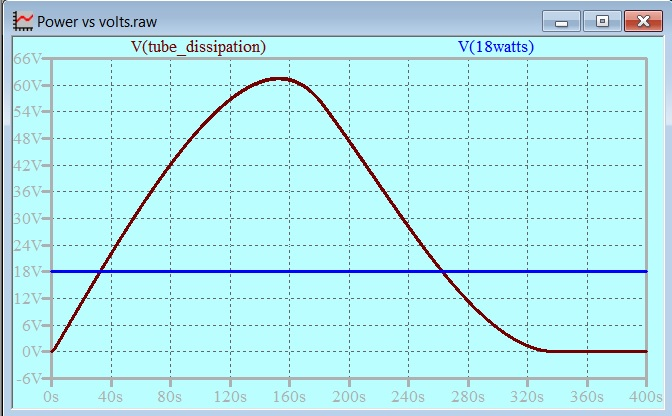Note that the vertical scale has a “V” after the number. This is volts as far as LTspice is concerned, but my model makes this voltage represent power. The unit is Watts. The horizontal axis is Load Voltage. Again, I varied this with time in my model, and LTspice has an “s” for seconds after the numbers. The units are volts.

If the supply were DC, this would be a parabola, but it is distorted as when the voltage rises, the proportion of the time that the bridge diodes are conducting is reduced. The maximum power point is where the voltage is about half the mains voltage as we would expect. Eighteen watts is drawn when the voltage at the rectifier output is either 32.69 volts or 262.55 volts. What’s the bet that the lower voltage is chosen! Either way, the light-fitting inductor is a critical component in the circuit. In a different posting, a mate of Dave Jones’ tells us that the inductor can be eliminated (shorted) to reduce losses. I DON’T THINK SO!
1. It looks to me that the impedance of the ballast is a key component and that it works in conjunction with a shunt regulator to determine the 18 watts dissipation.
2. Even if point 1. (above) were wrong, an ordinary switch mechanism has trouble working a room full of fluoro tube fitting power factor correction capacitors. It would vapourize if closed at mains peak with a load of many paralleled rectifier/res-cap combinations!

After the reservoir caps, there is a regulator, that Dave identifies as a buck regulator. On his video, Dave applies a current clamp to the LED array load and shows us the waveform.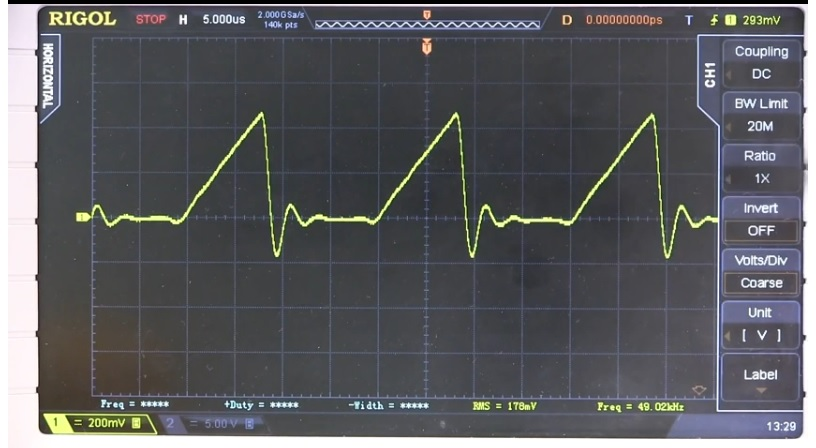He provides commentary to go with this waveform. He says “You can see the current ramp here, with a whole lot of ringing at the bottom of it.” Hmmmmm! I am not sure that I reckon that Dave’s comments are adequate in the face of the interesting details we see on his oscilloscope.

If Dave is right, and this is a buck regulator, then it is running in discontinuous inductor current mode. Discontinuous current can sometimes be just a waste of time – the circuit is not transferring energy when the current is not flowing. However in a lighting application this might not be so. Some say that the human eye responds to the peak brightness in a varying waveform. If we create subjective brightness at the peaks, then perhaps it is just a waste of energy to maintain brightness between peaks. This is an interesting subject that I will save for another day.

The thing about inductors (when not saturating) the rate of change of current is proportional to the voltage across the inductor. In a buck regulator, this means that the rate of rise of inductor current is proportional to the volt difference between the input voltage and the output voltage. When the switch is off, and the freewheeling diode is conducting, the rate of fall of inductor current is proportional to the output voltage with the same constant of proportionality.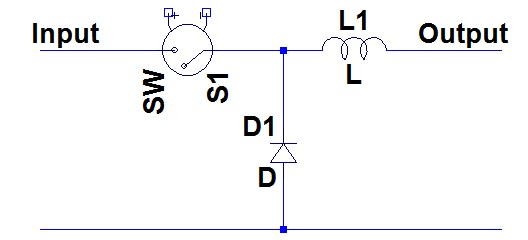This is the Buck Regulator TopologyThis is Buck!

We can obtain good estimates of the rates of change of current graphically from the oscilloscope picture.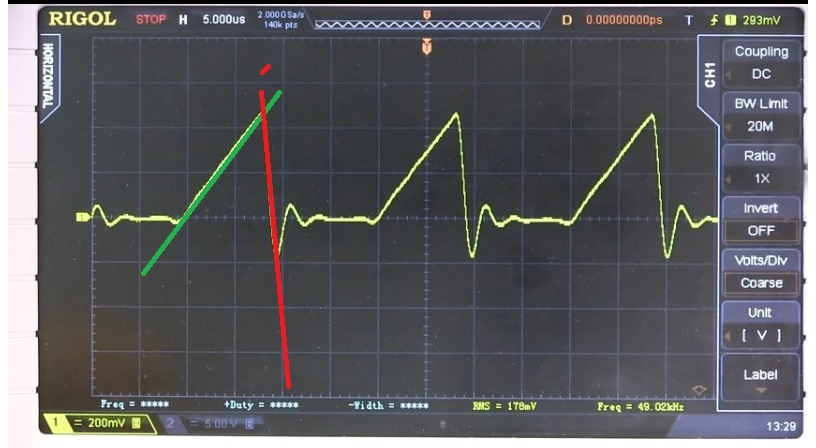The green line (Switch ON) rises 4 divisions in three time divisions.
The red line (freewheeling diode conducting) falls 5 divisions in half a division of time. Dave tells us that there are four strings of 24 LEDs. These LEDs have a forward volt drop of about 3 volt. That gives us 72 volts supply to the LEDs. A little algebra with the rates of change of voltage gives us:
Input voltage to buck regulator = 82.26. Of course this input volts to the buck regulator is the same as the output volts of the bridge rectifier that we were considering earlier. But this is the wrong voltage, isn’t it?

I cannot be confident that I have the inductance value of the ballast any closer than within an octave.

If the inductor is 600mH, then the low voltage for 18 Watts is 16.54 volts
If the inductor is 2400mH then the low voltage for 18Watts is 66.97 volts
If the inductor is 3000mH then the low voltage for 18 watts is 86 volts.

Maybe that is it. Dave Jone’s observation about the LED strings might be perfectly correct, and the ballast is actually about 3H.

There is more mystery yet. What is that ringing on the load current waveform when the inductor current ceases? I can simulate it. Compare these: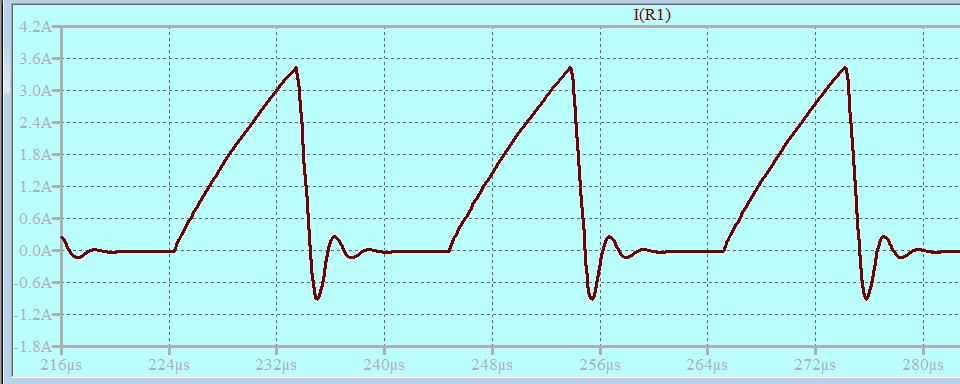Not a bad match. What worries me about it is that the damped ring cannot be sustained without a path going somewhere from the switch end of the inductor. When that ring is occurring, both the switch and the freewheeling diode are not conducting. To get the simulated waveform, I had to place 12nF across the switch, a most unlikely circuit feature.

Dave Jones has teased us here with more questions than he had answered. I see the buck converter working as a shunt regulator in conjunction with the high input impedance provided by the ballast. There was no hint of this in his analysis. He is pitching at a viewership that is quite different from this blog’s readership. I hope that neophytes don’t mistake his superficiality for the real thing.